# Mathematics Quiz For RRB NTPC : 14th January 2020 of Simple interest, ratio and Percentage

Q1. 117²+83²= ?
(a) 20698
(b) 20578
(c) 20798
(d) 20268

Q2. The ratio of two numbers is 3 : 4 and their H.C.F. is 4. Their L.C.M. is:
(a) 12
(b) 16
(c) 24
(d) 48

Q3. The population of a town increased from 1,75,000 to 2,62,500 in a decade. The average percent increase of population per year is:
(a) 4.37%
(b) 5%
(c) 6%
(d) 8.75%

Q4. If a sum of money at simple interest doubles in 12 years, the rate of interest per annum is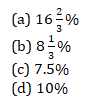Q5. Albert invested an amount of Rs. 8000 in a fixed deposit scheme for 2 years at compound interest rate 5 p.c.p.a. How much amount will Albert get on maturity of the fixed deposit?
(a) Rs. 8600
(b) Rs. 8620
(c) Rs. 8820
(d) None of these

Q6. By selling 100 pencils, a shopkeeper gains the selling price of 20 pencils. His gain per cent is
(a) 15%
(b) 20%
(c) 22%
(d) 25%

Q7. If 30% of (x + y) = 50% of (x – y), then x : y = ?
(a) 4 : 1
(b) 7 : 5
(c) 7 : 3
(d) 7 : 8

Q8. The ratio between the speeds of two trains is 7 : 8. If the second train runs 400 kms in 4 hours, then the speed of the first train is:
(a) 70 km/hr
(b) 75 km/hr
(c) 84 km/hr
(d) 87.5 km/hr

Q9. 0.04×0.0162 is equal to: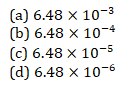Q10. Find the area of a right-angled triangle whose hypotenuse and base are 13 and 5 respectively?
(a) 26
(b) 24
(c) 18
(d) 30

Solutions: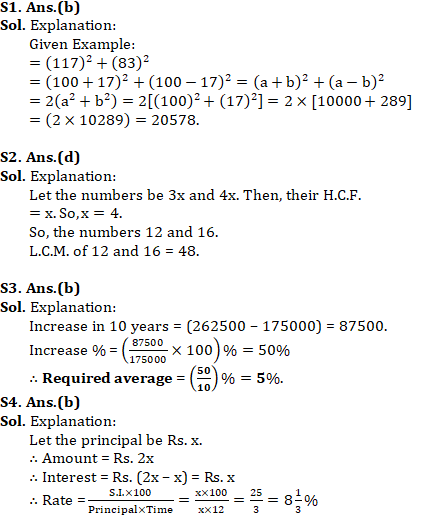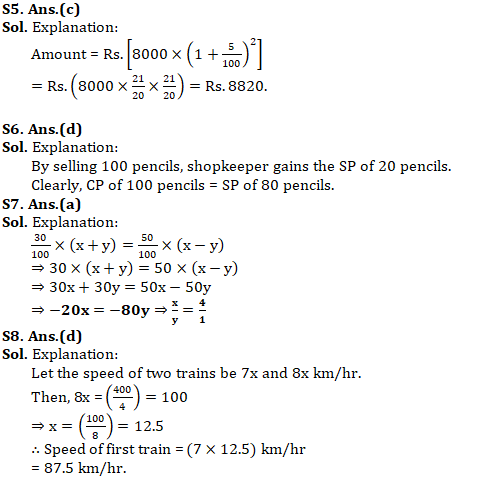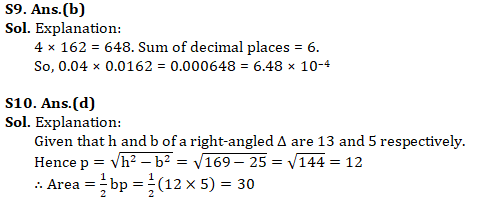Important Links for RRB NTPC Recruitment 2019